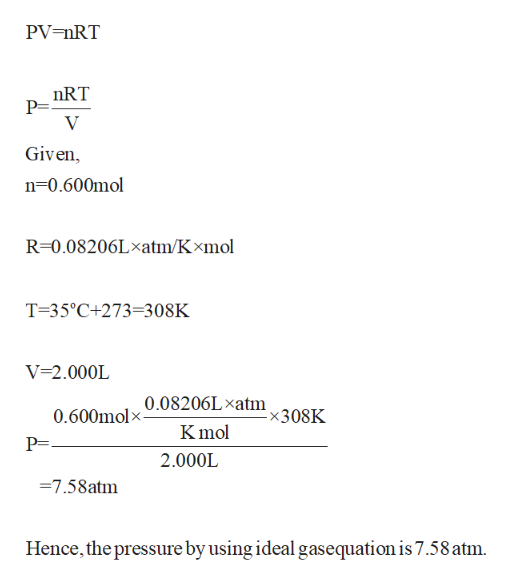# Calculate the pressure exerted by 0.600mol of N2 in a 2.0L vessel at 35oC using a) the ideal gas equation, b) the Van Der Waals equation

Question
15 views

Calculate the pressure exerted by 0.600mol of N2 in a 2.0L vessel at 35oC using a) the
ideal gas equation, b) the Van Der Waals equation

check_circle

Step 1

The pressure can be calculated via ideal gas la...help_outlineImage TranscriptionclosePV nRT nRT P= V Given n-0.600mol R-0.08206Lxatm/Kxmol T-35°C+273=308K V-2.000L 0.08206Lxatm x308K 0.600molx- Kmol P= 2.000L 7.58atm Hence, the pressure by using ideal gasequation is 7.58 atm. fullscreen

### Want to see the full answer?

See Solution

#### Want to see this answer and more?

Solutions are written by subject experts who are available 24/7. Questions are typically answered within 1 hour.*

See Solution
*Response times may vary by subject and question.
Tagged in

### Chemistry